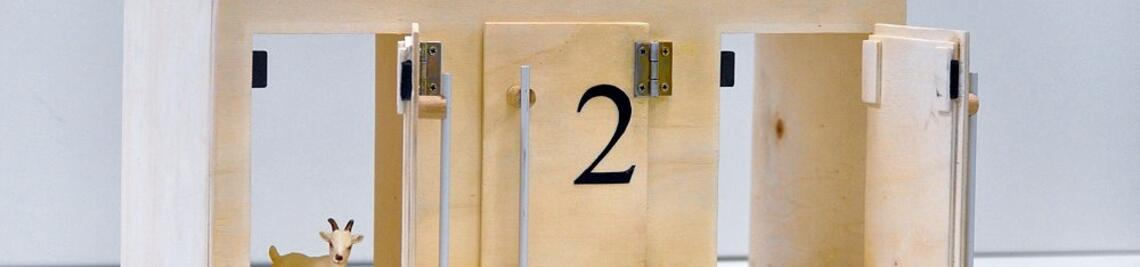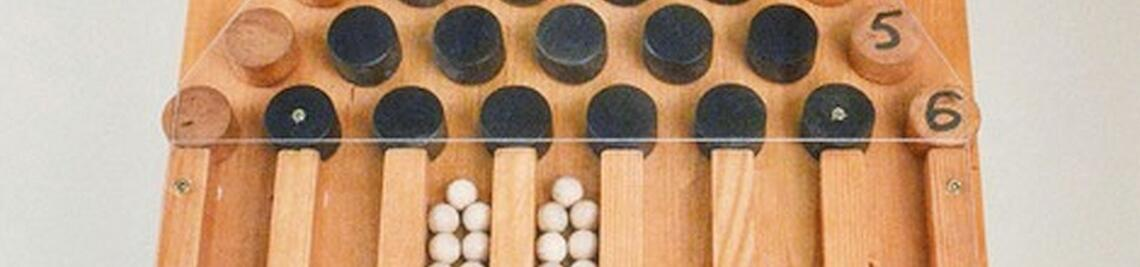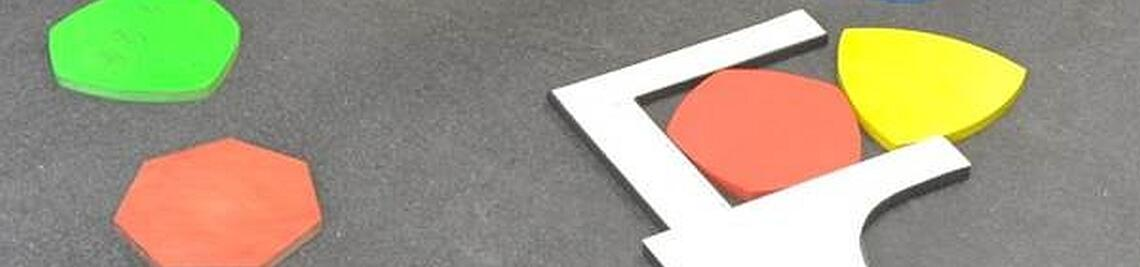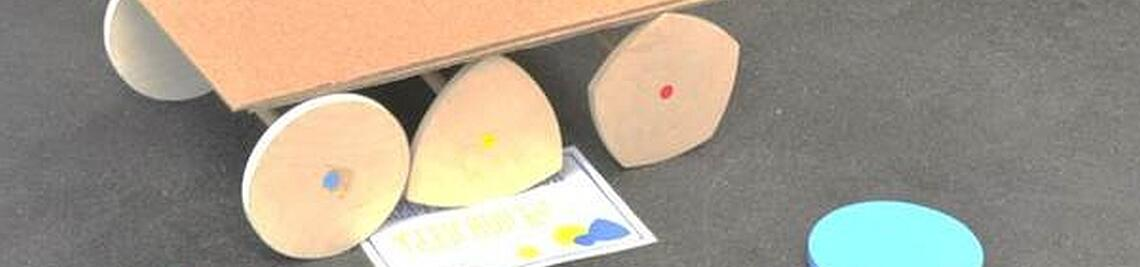••••# School meets Modelling

Real existing processes can be described with the help of mathematics, so that suitable questions can be answered. These questions can address for instance the development of the process or properties of the examining facts. The implementation and analysis of these questions is certainly of interest for  schools students, in order to solve real problems.

In reality, there are tasks that are characterized by a variety of cross-references to other departments such as computer science, biology and physics. The importance of mathematics as a means of gaining knowledge about the processing of such tasks can be experienced in this way. Modeling tasks combine all these aspects, as real problems are mathematized, solved by mathematical methods, and the results obtained are validated and interpreted again taking into account reality. Such tasks are not about finding the right solution; rather, modeling tasks are characterized by a multitude of possible strategies to reach a solution.

Duration: several school days

Contact: Hans-Stefan Siller